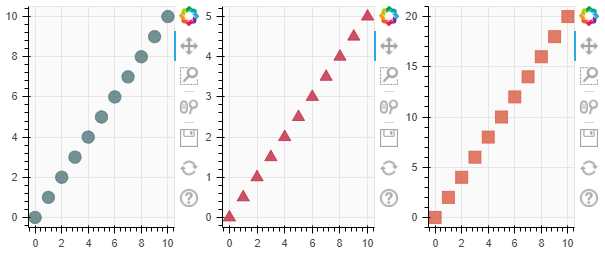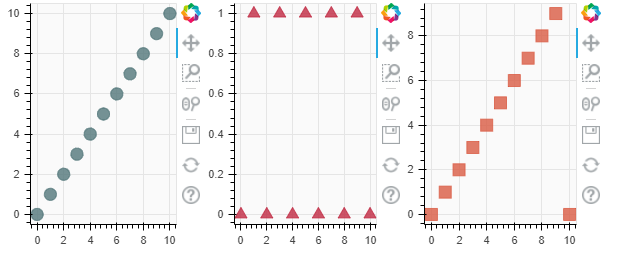Skip to content
Related Articles
Bokeh – Horizontal layout of plots
• Last Updated : 25 Feb, 2021

Bokeh includes several layout options for arranging plots and widgets. They make it possible to arrange multiple components to create interactive dashboards or data applications. The layout functions let you build a grid of plots and widgets. You can nest as many rows, columns, or grids of plots together as you’d like. In addition, Bokeh layouts support a number of “sizing modes”. These sizing modes allow plots and widgets to resize based on the browser window.

In Bokeh Row layout all the plots will be shown in a row only. This can be done using the row layout function supported under Bokeh:

Syntax:

row( plot1, plot2, …. , plotn)

Approach:

• Import module
• Create data
• Normally create multiple plots as if they are independent to each other.
• Combine them in one layout using rows()
• Display plots

Example:

## Python3

 `# python program for bokeh row layout``from` `bokeh.io ``import` `output_file, show``from` `bokeh.layouts ``import` `row``from` `bokeh.plotting ``import` `figure`` ` `# output will be in output.html``output_file(``"output.html"``)`` ` `x ``=` `list``(``range``(``11``))``# y will be same as x``y ``=` `x``# y0 divide every element of x by 2``y0 ``=` `[i``/``2` `for` `i ``in` `x]``# y1 multiply every element of xby 2``y1 ``=` `[i``*``2` `for` `i ``in` `x]`` ` `# now creating three plots``plot1 ``=` `figure(plot_width``=``200``, plot_height``=``250``, background_fill_color``=``"#fafafa"``)``plot1.circle(x, y, size``=``12``, color``=``"#53777a"``, alpha``=``0.8``)`` ` `plot2 ``=` `figure(plot_width``=``200``, plot_height``=``250``, background_fill_color``=``"#fafafa"``)``plot2.triangle(x, y0, size``=``12``, color``=``"#c02942"``, alpha``=``0.8``)`` ` `plot3 ``=` `figure(plot_width``=``200``, plot_height``=``250``, background_fill_color``=``"#fafafa"``)``plot3.square(x, y1, size``=``12``, color``=``"#d95b43"``, alpha``=``0.8``)`` ` `# now creating row layout``show(row(plot1, plot2, plot3))`

Output :Example:

## Python3

 `from` `bokeh.io ``import` `output_file, show``from` `bokeh.layouts ``import` `row``from` `bokeh.plotting ``import` `figure`` ` `output_file(``"output.html"``)`` ` `x ``=` `list``(``range``(``11``))``# y0 is same as x``y0 ``=` `x``# y1 is every element of x %2``y1 ``=` `[i ``%` `2` `for` `i ``in` `x]``# y1 is every element of x %10``y2 ``=` `[i ``%` `10` `for` `i ``in` `x]`` ` `plot1 ``=` `figure(plot_width``=``200``, plot_height``=``250``, background_fill_color``=``"#fafafa"``)``plot1.circle(x, y0, size``=``12``, color``=``"#53777a"``, alpha``=``0.8``)`` ` `plot2 ``=` `figure(plot_width``=``200``, plot_height``=``250``, background_fill_color``=``"#fafafa"``)``plot2.triangle(x, y1, size``=``12``, color``=``"#c02942"``, alpha``=``0.8``)`` ` `plot3 ``=` `figure(plot_width``=``200``, plot_height``=``250``, background_fill_color``=``"#fafafa"``)``plot3.square(x, y2, size``=``12``, color``=``"#d95b43"``, alpha``=``0.8``)`` ` `show(row(plot1, plot2, plot3))`

Output :Attention geek! Strengthen your foundations with the Python Programming Foundation Course and learn the basics.

To begin with, your interview preparations Enhance your Data Structures concepts with the Python DS Course. And to begin with your Machine Learning Journey, join the Machine Learning – Basic Level Course

My Personal Notes arrow_drop_up